# Energy and Thermodynamics

#### By Mark Ciotola

First published on June 15, 2019. Last updated on February 13, 2020.

# Energy and Thermodynamics

### By Mark Ciotola

First published on June 15, 2019. Last updated on February 13, 2020.

1. ## 1 Thermodynamics

First published on May 16, 2019. Last updated on February 15, 2020.

## Introduction

Thermodynamics is a branch of physics that concerns the flow of heat energy and the ability to convert energy into work. (Work is also expressed as force x distance). Thermodynamics is extensively used in chemistry, atmospheric science, geology and engineering.

Thermodynamics is part of a yet larger branch of physics called statistical mechanics, which bridges thermodynamics with modern physics. In fact, the discovery of quantum mechanics was an outcrop of thermodynamics. Josiah Williard Gibbs first utilized a quantum approach to express chemical reactions. Max Planck then utilized a quantum approach to express energy levels in photons being emitted from a hot object.

## Motivation and Applications

An important motivation for development of thermodynamics as a discipline was to understand how to make engines more efficient, and what the maximum efficiency for engines could be. Another application was to express the energy involved in chemical reactions. Today, thermodynamics is useful for a wide range of applications from energy efficiency to economics.

2. ## 2 Introduction to Energy

First published on . Last updated on February 6, 2021.

## Popular Definition of Energy

When most people refer to energy, they mean the amount of energy in a substance such as coal or petroleum that can be transformed into useful work. For example, a liter of gasoline can be combusted to power an automobile to drive uphill for several kilometers. Or, they are referring to electric energy, which can likewise be used to perform work.

## Physics Definition of Energy

The physics definition of energy includes the popular definition, but it is broader. In physics, energy can also refer to such that cannot be used but is nevertheless present. For example, ambient heat contains energy. Likewise, there is tremendous nuclear energy contained in the atoms comprising ordinary household objects such as forks and spoons but which cannot be accessed for useful purposes.

• The  physics definition of energy $$E$$ refers to the quantity of motion in a system, where $$m$$ is the mass of an object and $$v$$ is its velocity.

$$E = \frac{1}{2}mv^2$$

Energy can also refer to stored energy. Energy occurs in several forms:

• Moving objects possess kinetic energy
• Batteries, springs and raised objects store potential energy. For example, the energy stored in a spring, where $$x$$ is the distance a spring is compressed or stretched, and $$k$$ is a constant of proportionality, is:

$$E =kx^2$$

• Randomly-moving molecules represent heat energy
• Molecular bonds can store chemical energy
• Atomic nuclei can store nuclear energy

## Units of Energy

There are several units in which energy can be expressed.

• The standard scientific unit is the Joule, or $$J$$.
• A less standard science unit is the calorie, or $$cal$$. One calorie is the amount of energy required to raise one gram of water by one degree Celsius at standard atmospheric pressure.
• In the USA, the unit for energy in food is also called the Calorie (with a capital C), but the food Calorie $$Cal$$ is equal to 1000 science calories.
• The energy involved in heating systems is often expressed as in terms of the British Thermal Unit, or $$Btu$$. One Btu comprises about 1055 Joules.
• Electric energy, such as that delivered to your home, is often expressed in terms of the kilowatt-hour $$kWh$$. One Watt, $$W$$, is equal to one Joule per second. The Watt is a unit of power. Multiplying power by time results in an expression of energy.Photovoltaic panels (source: U.S. Dept. of Energy)

## Power

As mentioned, when most people refer to energy, they mean useful energy, for example a quantity of coal or petroleum. However, when people want to use energy, they nearly always mean power, which is how much work (essentially useful energy) can be delivered per unit of time. Watts, KiloWatts, and Horsepower are units of work.

## Production of Power

Power can be produced by many means. Animals such as horses convert foodstuffs such as hay into muscular force that can be used to pull carts and plows. Wood or coal can be turned to create a thermal difference vis-a-vis the atmosphere that can be used to drive steam engines. Petroleum can drive combustion engines. Photovoltaic cells can convert high energy solar photons into electrical power. Wind mills convert the pressure differentials manifested by  wind into electrical motion.

3. ## 3 First Law of Thermodynamics

First published on . Last updated on April 30, 2021.

## The Law

The First Law of Thermodynamics states that the total energy of an isolated system shall not change. In other words, the First Law of Thermodynamics requires that energy can neither be created nor destroyed. In other words, energy is conserved. This simply means that if heat flows from one object to another, the quantity of heat leaving the first object must equal the quantity of heat entering the second object.

## Discussion

The total energy shall neither increase more decrease. In physics, we say that the energy of an isolated system is conserved. (In physics, the term conserved has nothing to do with the environmental term of conservation).

What is an isolated system? Not surprisingly, a characteristic of isolated systems is that energy can neither enter or leave them. For example, a perfectly well-insulated container of hot water would be an isolated system. Although that particular hot water system is impossible in real life, it can be fairly well approximated using a reflective vacuum chamber, such as in an old-style coffee thermos. The entire Universe is considered to be an isolated system.

## Mixing example

A simple example that demonstrates the First Law is to mix a quantity of cool water with an equal quantity of hot water. If the water is kept in insulated containers before and after the mixing, then the temperature of the final mixture will be the mean of the temperatures of the original constituents (there may be a slight variation due to evaporation or escaped heat). In other words, the total amount of heat energy remained the same despite the mixing and temperature changes.

## Conduction example

Another simple example concerns a thermal conductor through which thermal energy flows from a warmer body of to a cooler body. The rate of thermal energy transfer Q is known as Newton’s Law of Cooling. We call the bodies reservoirs. The “hot” reservoir has a temperature of TH, and the “cold” reservoir has a temperature of TC. The conductor is of area (cross section) A, and of length L.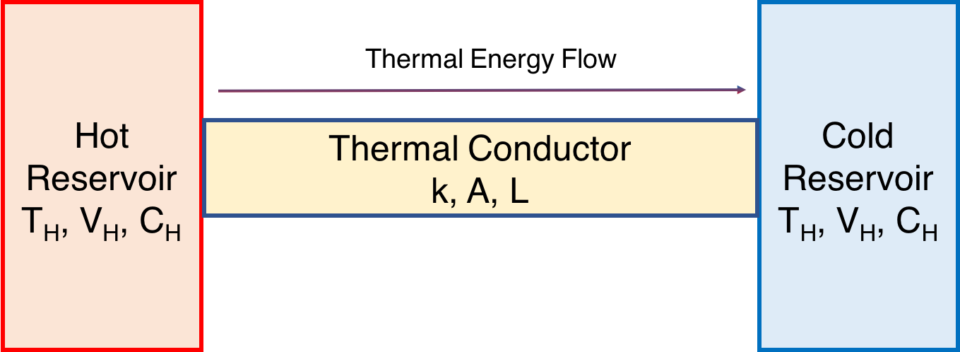Conductor bridging a thermal difference

The flow of thermal energy is:

$$\Large{\frac{dQ}{dt}}=kA\frac{T_H – T_C}{L}$$.

The quantity of heat energy lost by the warmer body is identical to the quantity of heat energy gained by the cooler body. This example can be easily replicated by using a U-shaped aluminum conductor to bridge two well-insulated cups of water of different temperatures.(The conductor should be appropriately insulated as well for best results).

Use the following simulation to try out Fourier’s Law. (The simulation may take a moment to load).

[iframe src=”https://heatsuite.herokuapp.com/fourier/start” width=”100%” height=”500″]

## Conversion of Form of Energy

The First Law allows energy to be transformed from one form into another, such as from potential to kinetic energy. Yet the total amount of energy must remain the same.

Consider the case of a swinging pendulum (in a vacuum). As the pendulum bob falls, it will speed up, and its kinetic energy shall consequently increase, while its potential energy (due to gravity) shall decrease. When the bob rises, its potential energy increases at the cost of its kinetic energy. In all cases, though, the total energy remains the same:

$$Total~Energy = Kinetic~Energy+Potential~Energy+Thermal~Energy$$.

Likewise, there is no change in the total energy of the system:

$$\Delta T + \Delta V + \Delta Q = 0$$,

where

$$\Delta T$$ is the change in kinetic energy, $$\Delta V$$ the change in potential energy, and $$\Delta T$$ the change in thermal energy.

What if we now have the pendulum operate in air, instead of a vacuum? The pendulum will gradually slow down due to air resistance. The total of potential + kinetic energy shall decrease! However, according to the First Law, the energy must go somewhere. It cannot merely disappear. As friction continues to operate and the pendulum continues to hit air molecules, the average (mean) velocity of the individual air molecules increases. So the air heats up a bit, and the pendulum’s energy is gradually transferred into heat energy. Eventually, the motion of the pendulum will stop, but energy in the air will have increased, as exhibited by an increased air temperature.

## Modern Physics Modification

Who has not heard of Albert Einstein’s famous equation $$E = mc^2$$? Energy can be changed into mass and vice-versus. So the First Law must be modified to take into account this mass-energy equivalence. This is the realm of nuclear reactors and nuclear bombs, and its effect is insignificant on most systems we encounter in our lives.

## Resources

• Paper concerning Fourier’s Law of Thermal Conduction
• Paper concerning Newton’s Law of Cooling and a simulation.
• Newton’s Law of Cooling simulation.
• Newton’s Law of Cooling program code.

## Notes & References

The phrase “conservation of energy” has a much different meaning than the common phrase “conserving energy”. The latter refers to consuming less of useful forms of energy such as coal or petroleum.

Such demonstration kits are commonly sold by science education equipment firms. If ice water is used, then energy due to the phase change of melting ice must also be accounted for.

4. ## 4 Temperature

First published on . Last updated on April 29, 2021.

## Introduction

Recall that one form of energy is thermal energy, which comprises the random motion of individual molecules that are part of a larger system. For example, water molecules in a tea kettle are moving back and forth in all directions and at many different speeds. Some water molecules might be moving slowly, and they would have a lower energy than those that are moving quickly.

Temperature refers to the intensity of thermal energy. Regardless of the energy of individual molecules, if their average (mean) velocity is high, then the temperature of the collection of those molecules is high. The the average is low, then the temperature is low.

## Measuring Temperature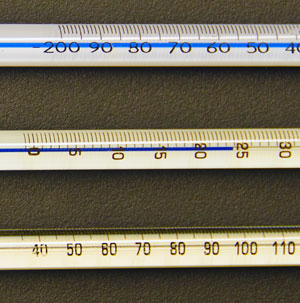Analog thermometers (source: U.S. govt.)

One cannot easily measure the individual velocities of molecules within a large collection of such. Fortunately, there exists easier ways to measure temperature. The traditional device for measuring temperature is the thermometer. Thermometers can operate by measuring the expansion of a fluid such as mercury or alcohol. Other thermometers operate by comparing the expansion on one metal to another.

More modern devices can measure temperature by detecting infrared radiation emitted from an object. Such devices only measure surface temperature, but adjustments can be made to infer the internal temperature of an object such as a human.

## Units of Temperature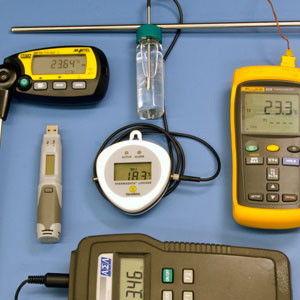Temperature measuring devices (source: U.S. government)

• In the USA, the degree Fahrenheit, F, is used to express temperature.
• In the metric system, the degree Celsius (or Centigrade)  expresses temperature.
• The preferred unit by physicists is the Kelvin, K. One unit of Kelvin is equal to one degree Celsius, except that the Kelvin system starts at absolute zero temperature. 0 degrees Celsius is equal to 273.15 Kelvin.

## Distinction Between Thermal Energy and Temperature

Thermal energyis the kinetic energy contained in the random movement of molecules. Temperature is the measure of the strengthor intensitysuch thermal energy. Temperature does not concern the amount of thermal energy. For example, a cup of water may be quite hot, yet contain much less thermal energy than the near-freezing Arctic Ocean. The relationship between temperature Tand thermal energy Qis as follows:

$$T = \frac{Q}{MC}$$,

where Mis the mass under consideration and Cis the heat capacity of the substance containing the thermal energy.

## Resource

U.S. National Institute of Standards and Technology (NIST), SI Units: Temperature.

5. ## 5 Second Law of Thermodynamics

First published on . Last updated on May 19, 2020.

## The Law

The Second Law of Thermodynamics states that the entropy on an isolated system can never decrease. More specifically,

$$\frac{dS}{dt} ≥ 0$$,

where $$S$$ is entropy and $$t$$ is time.

## Definition of Entropy

Our discussion of entropy shall pertain to physical entropyrather than information entropy. The two concepts are related but differ in ways that are beyond this discussion.

A rough rule of thumb is that entropy is what increases in real life systems when heat flows, pressures equalize or substances become more mixed. For example, when you let the air out of a tire or balloon, and you hear the hiss as the compressed air escapes, entropy is increasing.

With the advent of modern physics, the term entropy $$S$$ has a precise definition. It is Boltzmann’s constant $$k$$ multiplied by the log of multiplicity $$\Omega$$.

Boltzmann’s~constant: $$k = 1.381 x 10^{-23} m^2~kg~s^{-2}$$.

Entropy: $$S = k \ln{\Omega}$$.

### Multiplicity

Multiplicity is the number of ways a state can be produced in a given system. For example, in a system of three US pennies, a state comprising two heads can be comprised three ways:

[table]

Coin 1, Coin 2, Coin 3

H, H, T

H, T, H

T, H, H

[/table]

Hence, the multiplicity of this system is three.

### A Few More Points

For entropy calculations, we are typically considering how the instances of energy can be arranged, but types of particles also have an effect.

It is important to note that the Second Law does not prohibit the entropy of a system from increasing.

## Ways Entropy Increases

There are commonly several situations where entropy increases. When heat flows result in no work, or less than the Carnot ideal, entropy increases. Many chemical reactions result in increases of entropy, such as when gasoline is combusted to propel an automobile. Entropy is increased when substances become more mixed even where no chemical reaction occurs, such as when helium and neon gasses become mixed together.

## Cosmological Perspective

The Second Law requires that the total entropy of the universe must increase over time.Yet, the expansion of the universe result in decreasing, not increasing, mean entropy density of the universe. These two trends are not inconsistent. Total entropy of the universe is indeed increasing, but it is being spread out more quickly than it increases.

Yet, locally, gravity pulls together matter and produces local regions of higher entropy such as stars and planets. So really, there are several contrasting trends. The total entropy of the universe increases. Yet as the universe expands, the mean entropy density decreases. Nevertheless, locally, gravity may result in local clumps of high entropy. Then, eventually the entropy of those clumps dissipates into the surrounding universe.

## Notes & References

Such a trend extrapolated into the distant suggests that the universe will die a classic heat death, in which no work or life is possible.

As long as this continues to be the case, reports of the universe’s impending heat death may be greatly exaggerated, or at least further off than once thought.

6. ## 6 Heat Engines

First published on . Last updated on April 29, 2021.

## Introduction

A system that has pure physical aspects as well as social aspects is that of the engine. The Second Law of Thermodynamics tells that even the best real life heat engines will produce entropy along with work. No engine can produce work alone. (Thermal conduction itself results in lots of entropy production but little work).

The heat engine is a common example used to illustrate the Second Law of Thermodynamics. A heat engine utilizes a temperature difference (a thermodynamic potential) to perform work, such as moving a train or pumping water.A heat engine

A simple heat engine is shown here. Most technical details have been omitted. Here, a tank of water that is presumably heated into high pressure steam by a flame or other energy source. The tank contains a piston that converts some of that heat energy into a cyclic in-out motion that represents work done upon a load, here represented by the wheel. The little puffs of steam represents the waste heat.

## How A Heat Engine Functions

For a heat engine to function, heat must flow across a temperature difference from a warmer region to a cooler one. Such regions are called thermal reservoirs. Warmer heat reservoirs can be flames, hot air or steam, for example. Cooler heat reservoirs can be ice, cold air or cool water, for example. Air at room temperature can serve as either kind of reservoir depending on how hot or cold the other reservoir is.

The warmer region is typically called the hot reservoir (regardless of its actual temperature) with temperature $$T_H$$, while the cooler region is designated as the cold reservoir with temperature $$T_C$$. A typical heat engine undergoes a cycle of actions where heat flows into the engine, increases pressure of a fluid such as air or steam, used that pressure to push a piston, then releases the heat whereupon pressure is reduced. 

## Example

The redder and higher the warmer heat reservoir, the hotter is it. Conversely, coolness is represented by bluer shading and lower height. The bluer and lower the cooler heat reservoir, the colder it is.  Our heat engine begins operating between a quite hot and a quite cold reservoir as shown here.

Here we see a heat engine working between a warmer reservoir and a cooler reservoir (below). An example of this scenario is the temperature difference between a hot flame and a cool tank of water being used in a steam engine. When thermal energy flows to power a heat engine, part of the available energy is put into work and the remainder results in waste heat.

Below, the lefthand red area represents the hot reservoir, and the righthand blue area represents the cold reservoir.  Warmth is represented by redder shading (if your version is in color) and greater height. The gray area represents the engine. Heat flows through the engine, which does work by turning a wheel, which might drive a generator, push a car or run a machine tool.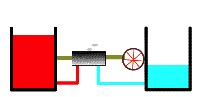Heat engine operating upon a thermal potential

## Efficiency

No engine turns allof the heat flow into work. That would imply 100% efficiency, which is impossible in theory as well as practice, regardless of how well the engine is constructed. The Second Law of Thermodynamics tells that even the best engines will produce entropy along with work. Efficiency $$\epsilon$$can be expressed as:

$$\epsilon = \frac{Q_H – W}{Q_H}$$

where Q_His the flow of thermal energy out of the hot reservoir and Wis work.

### Carnot Efficiency

The best efficiency that an ideal engine can achieve is known as its Carnot Efficiency. The efficiency of a Carnot Engine depends upon the temperature difference between the thermal reservoirs, where the temperature of the hot reservoir is Thand that of the cold reservoir is Tc. The Carnot Efficiency $$\epsilon_c$$ is simply the difference between the warmer and cooler temperature divided by the warmer temperature:

$$\epsilon_c = 1 – \frac{T_C}{T_H}$$

Calculating the Carnot Efficiency must be done using absolute temperatures, that is, temperatures measured from absolute zero. Absolute zero is the lowest possible temperature in theory, and has never been quite obtained in practice. Such temperatures are measured in a kind of degree called Kelvin. 0° Celsius equals about 273.15 Kelvin.

An example is the temperature difference between a hot flame and a cool tank of water being used in a steam engine. Then, part of the available energy is used to perform work and the remainder is exhausted as waste heat. For instance, a steam engine could contain a piston that converts some of the heat flow into a cyclic in-out motion that represents work done upon a load, such as a flywheel wheel. Steam released into cooler air represents waste heat. When waste heat is created, an intangible quantity called entropy is produced. The more the heat engine works, the more entropy it will produce.

## Work Performed by a Carnot Engine

If an amount of heat $$Q_H$$ is removed from the hot reservoir, the the amount of work $$W$$ a Carnot engine will perform is

$$W = \epsilon Q_H$$.

## Work Performed by Real Life Heat Engines

In reality, most engines are a great deal less efficient than even the Carnot efficiency. While a Carnot Engine can be approximated in real life, despite its high efficiency, it functions too slowly to be of much value for real life uses. Practical heat engines tend to operate of much lower efficiencies. This real life efficiency is called “Second Law Efficiency”, $$\epsilon_s$$.

$$\epsilon_{Second~Law} \geq \epsilon_{Carnot}$$.

One reason that engines operate at lower efficiencies than better construction would allow is that more efficient heat engines tend to take more resources to build.

## Further Discussion

If heat flows from a warmer object to a cooler object (where no engine is involved), no work results, but entropy is still produced (or you could say that the entropy of the system under consideration increases). Thermal conduction itself results in lots of entropy production but little work. A thermal conductor can be thought of as a lazy heat engine. Chemical reactions, such as burning coal and oil or metabolizing sugars also results in entropy production. The Second Law of Thermodynamics states that overall entropy (of an entire system) will tend to increase.

## Notes & References

 For the origins of heat engine theory, read the works of Sadi Carnot.

In theory, a heat engine is not required to produce entropy if the temperature of the cold region is absolute zero (which is about – 273° C). In practice, such a low temperature is physically impossible.

7. ## 7 Heat Engine with Exhaustible Reservoirs

First published on . Last updated on April 29, 2021.A heat engine

Recall that heat engine utilizes a temperature difference (a thermodynamic potential) to perform work Here we see a heat engine working between a warmer reservoir and a cooler reservoir (below). Warmth is represented by redder shading (in your version is in color) and greater height. The redder and higher the warmer heat reservoir, the hotter is it. Conversely, coolness is represented by bluer shading and lower height. The bluer and lower the cooler heat reservoir, the colder it is.  Our heat engine begins operating between a quite hot and a quite cold reservoir as shown here.Heat engine operating upon “full” thermal reservoirs

As the heat engine continues to operate, the warmer heat reservoir becomes less hot and the cool reservoir becomes less cold (below).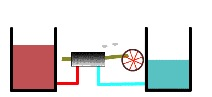Thermal reservoirs partially depleted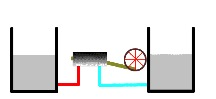Thermal reservoirs completely depleted

Eventually, both the warmer and cooler heat reservoirs reach the same temperature (see figure). When this happens, no more work is possible. The heat engine is no longer operable. At this point, the reservoirs are said to be in thermal equilibrium.

8. ## 8 The Firm As A Heat Engine

First published on May 11, 2021. Last updated on May 11, 2021.

The firm is at the heart of microeconomics. For a firm to thrive, it must make a gross profit. Several of the concepts of firms and microeconomics are analogous to those concerning thermodynamics and heat engines.

For example, thermal energy removed from the hot reservoir $$Q_H$$ is analogous to revenue (R). Thermal energy exhausted into the cold reservoir $$Q_C$$ is analogous to cost (C).